Q

# Using four unequal sticks, as you did in the above activity, see if you can form a quadrilateral such that (a) all the four angles are acute

Using four unequal sticks, as you did in the above activity, see if you can form a quadrilateral such that

(a) all the four angles are acute.

(b) one of the angles is obtuse.

(c) one of the angles is right angled.

(d) two of the angles are obtuse.

(e) two of the angles are right angled.

(f) the diagonals are perpendicular to one another

Views

(a) all the four angles are acute.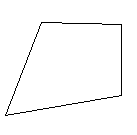(b) one of the angles is obtuse.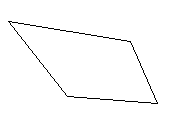(c) one of the angles is right angled.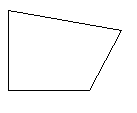(d) two of the angles are obtuse.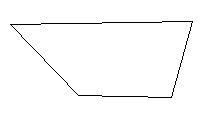(e) two of the angles are right angled.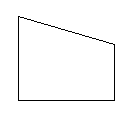(f) the diagonals are perpendicular to one another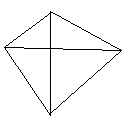Exams
Articles
Questions